Corporate Finance 2.01: Update to the first printing

Page number

In text currently

Correction

53

Cumulated Future Value = \$5,000(1.06)9+……. + \$5,000(1.06) =

Cumulated Future Value = \$5,000(1.06)9+……. + \$5,000(1.06) + \$5,000 =

62

As long as g is less than n…

As long as g is less than r….

120

Duration of a bond =

# No t in denominator

124

Price of Boeing bond =      = \$1400.45

Price of Boeing bond =      = \$1404.25

125

For instance, assume that Boeing has a cost of equity of 10.54%

Thus, for Boeing, the cost of equity is 10.54%

For instance, assume that Boeing has a cost of equity of 10.58%

Thus, for Boeing, the cost of equity is 10.58%

131

Value of Equity in Infinite-life asset = E(FCFEt)/ (ke-gn)

Value of Equity in Infinite-life asset = E(FCFE1)/ (ke-gn)

134

Value of high growth business =

Terminal value = …… = \$43,058

Denominator should be kc

Terminal value = …… = \$43,049

135

When you buy the right to sell an asset….

With a put option…., you buy the right to buy the asset at the current price and sell it back at the exercise price,

With a put option…., you buy the right to buy the asset at the exercise price and sell it back at the current price,

162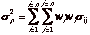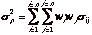169

E(R)    =          Rf + bj [E(Rj)-Rf] + b2 [E(R2)-Rf]  ...+ bn [E(Rn)-Rf]

E(R)    =          Rf + b1 [E(R1)-Rf] + b2 [E(R2)-Rf]  ...+ bn [E(Rn)-Rf]

191

only 9.54% (1.20.5-1=1.0954)

only 9.54% (1.20.5-1=.0954)

199

Figure 7.3

RAW BETA = 0.98

Figure 7.3

RAW BETA = 0.96

201

Jensen’s alpha=1.11%-0.4%(1-.62)= 1.01%

Annualized Jensen’s alpha = (1.01)12-1= 12.68%

Jensen’s alpha=1.11%-0.4%(1-.62)= .96%

Annualized Jensen’s alpha = (1.01)12-1= 12.12%

204

bL  = bu  (1 + (1-t) (D/E)

bL  = bu  (1 + (1-t) (D/E))

205

Unlevered Beta= 0.96 / ( 1 + (1 - 0.35)) (0.1788) = 0.86

Unlevered Beta= 0.96 / [( 1 + (1 - 0.35)) (0.1788)] = 0.86

247

In Table 8.9

Home Depot Expo     Debt ratio = 6.32%

In Table 8.9

Home Depot Expo     Debt ratio = 6.62%

272

·      The firm will have to incur an advertising expense….

Remove bullet point (there is no advertising expense)

277

·      Subtract out the changes in working capital. Since… the initial investment in working capital is \$ 2 million …. and 10% of revenues in year 1. The changes…10%

Subtract out the changes in working capital. Since… the initial investment in working capital is \$ 3.2 million …. and 8% of revenues in year 1. The changes…8%

287

Last line..

Geometric Average…. = 26.40%

Last line..

Geometric Average…. = 25.30%

288

After-tax Return on capital = \$ 270

\$1000

After-tax Return on capital = \$ 270/\$1000

298

In Practice 10.7

… the cost of capital of 9.38%

In Practice 10.7

… the cost of capital of 9.32%

300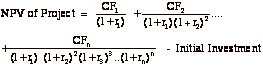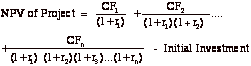326

D Exchange Rated/f =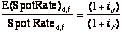D Exchange Rated/f =-1

329, 330

In last two paragraphs and table 11.8

\$0.6525

In last two paragraphs

\$0.65625

337

Table 11.10

Year 10 – Change in working capital (1955.02)

aFuture

Table 11.10

Year 10 – Change in working capital  +1955.02

aFree

354

= - \$3000 - \$30,000 [PV(A,10%,5 years)]

= - \$30,000 - \$3,000 [PV(A,10%,5 years)]

359

…the 5-year project yields \$37.98 more per year

…the 5-year project yields \$38.02 more per year

362

In Practice 12.7

Net Initial Investment in New Machine = …= \$135,000

Annual Tax Savings from Additional Depreciation on New Machine = Depreciation on old machine – Depreciation on new machine

In Practice 12.7

Net Initial Investment in New Machine = …= - \$135,000

Annual Tax Savings from Additional Depreciation on New Machine = Depreciation on new machine – Depreciation on new machine

368

CC 12.3:… required for project B was \$ 40 million.

CC 12.3:… required for project B was \$ 30 million.

372

# Table 12.7: Lost Sales are in units

376

In Practice 12.12

Table 12.8 summarizes after-tax operating income

In Practice 12.12

Table 9.6 summarizes before-tax operating income

377

Footnote 7: ….at 9.38%

Footnote 7: ….at 9.32%

378

Table 12.11

Year            …. PV of Incremental OI

3                         38,805

4                                      37,915

PV of Synergy    349,657

In text

NPV of Restauarant = -\$300,000 + \$128,623 +\$349,657 = \$178,280

Table 12.11

Year            …. PV of Incremental OI

3                         35,435

4                                      34,623

PV of Synergy    342,995

In text

NPV of Restauarant = -\$300,000 + \$128,623 + \$342,995= \$171,618

395

… at the project’s cost of capital of 9.38%

… at the project’s cost of capital of 9.32%

397

… since the working capital investment would be only 7%…

… since the working capital investment would be only 7.2%…

406

… with 99% probability

….. with 95% probability

410

In  Practice 13.10

…offers 30-day credit

In  Practice 13.10

…offers 1-year credit

444

Figure 14.5

Y Axis: Cash Balance (\$millions)

X Axis: Market Value of Equity (%)

Figure 14.5

Y Axis: Number of firms

X Axis: Cash/Market Value of Equity (%)

445

Footnote 8: … Boeing’s return on capital of 510% to its cost of capital of 9.23%

Footnote 8: … Boeing’s return on capital of 5.82% to its cost of capital of 9.17%

497

Table 16.2

3                   3639131

4                   3690108

5                   3744042

15               (10,510,869)

Table 16.2

3                   (3639131)

4                   (3690108)

5                   (3744042)

15               +10,510,869

506

5. … convertible bonds

9b. …. has 10,000 convertible bonds outstanding

5. …10-year semi annual convertible boinds

9b. …. has 10,000 convertible bonds outstanding, trading at par..

584

Effective tax rate = 613/1,751 = 30.05%

Effective tax rate = 613/2039 = 30.05%

586

Growth rate = (Firm Value * (Cost of Capital- FCFF/Firm Value + FCFF

= (40,789*.(0917-1,176)/(40,789+1,176) = .0611 or 6.11%

Growth rate = (Firm Value * Cost of Capital- FCFF)/(Firm Value + FCFF)

= (40,789*.0917-1,176)/(40,789+1,176) = .0611 or 6.11%

603

Value of Boeing as an Unlevered Firm

= \$40,789 million + \$2,868 million - \$32 million

Value of Boeing as an Unlevered Firm

= \$40,789 million - \$2,868 million + \$32 million

614

Problem 13

Problem 13

Riskfree rate is 7%.

629

Table 20.3

Footnote: Net Incomet-1

Table 20.3

Footnote: Net Incomet-1

631

Time Warner repays \$189 million

Time Warner repays \$190 million

670

Footnote 3: This is because 70% of intercorporate dividends are not raked.

Footnote 3: This is because 70% of intercorporate dividends are not taxed.

707

In Practice 22.8

Boeing = 0.3213 – 0.39023 (1.01) …. = 42.76%

The Home Depot = 0.3213 – 0.39023 (0.87) …. = 45.56%

In Practice 22.8

Boeing = 0.3213 – 0.39023 (0.87) …. = 48.22%

The Home Depot = 0.3213 – 0.39023 (1.01) …. = 40.09%

708

Boeing = 0.0190 – 0.01908 (1.01) …. = 1.97%

The Home Depot = 0.0190 – 0.01908 (0.87) …. = 2.09%

Boeing = 0.0190 – 0.01908 (0.87) …. = 2.23%

The Home Depot = 0.0190 – 0.01908 (1.01) …. = 1.82%

712

Problem 1

The firm’s current beta is 1.0 and the current T.Bond rate is 5.5%.

Problem 3

The firm has a cost of equity of 22%

Problem 1

The firm’s current beta is 1.0 and the current T.Bond rate is 8.5%.

Problem 3

The firm has a cost of equity of 16%

714

Problem 12

Problem 12

Add: Growth rate is 12% next year.

760

Cost of Capital = kequity (Equity/(Debt + Equity) +

Cost of Capital = kequity (Equity/(Debt + Equity)) +

777

… as a function of its profit margin, payout ratio, profit margin and expected growth

… as a function of its profit margin, payout ratio, profit margin and expected growth

780

Value of Equity = \$977,300 million X 1.40

Value of Equity = \$977.3 million X 1.40

781

Predicted PBVBoeing = …….. = 2.27

… is overvalued by roughly 35%.

Predicted PBVBoeing = …….. = 2.30

… is overvalued by roughly 54%.

783

We will use the results of the market regression summarized in Table 24.20

We will use the results of the market regression summarized in Table 24.22

791

Gary Condit

Philip Condit

794

Gary Condit

Mr. Condit

796

Figure 25.3

Y axis: Value (thoustands)

Figure 25.3

Y axis: Value (millions)

809

Figure 25.8

Terminal Value = 2298(.0842-.05)

Cost of equity = 13.05%

Figure 25.8

Terminal Value = 2298 / (.0842-.05)

Cost of Equity = 10.58%

810

Figure 25.9

Terminal Value = 6666 (.0792-.05)

Figure 25.9

Terminal Value = 6666 / (.0792-.05)

811

Firm’s D/E Ratio = 7.09%

Firm’s D/E Ratio = 25%

814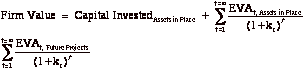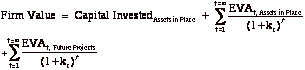826

… the relationship between EVA changes

… the relationship between CFROI changes

849

In Practice26.4

In Practice26.4

# Add: Depreciation in the current year is \$545 million

859

Figure 26.5: Synergy 2422 million

Figure 26.5: Synergy 2432 million

870

Table 26.5

Year         EBIT

1983         113.15

Table 26.5

Year         EBIT

1983         133.15

898

In Practice 27.4

… the present value of the expected cashflows from the store is 120 million FF

In Practice 27.4

… the present value of the expected cashflows from the store is 80 million FF

904

Value of Put = ……. = \$474,831

Value of Abandonment Option = \$ 474,831

NPV with abandonment option = - \$462,456

Value of Put = ……. = \$783,464

Value of Abandonment Option = \$783,464

NPV with abandonment option = - \$153,823

921

Problem 1

… growing at 5% a year until the patent expires

Problem 1

… growing at 5% a year forever

Solutions to problems

Check solutions on web site for any corrections